# Google Spreadsheet COUNTIF function with formula examples

Google Sheets COUNTIF is one of the easiest functions to learn and one of the handiest to use.

It's time to pick up some knowledge on how COUNTIF is used in Google Spreadsheet and learn why this function makes a true Google Spreadsheet companion.

## What is the COUNTIF function in Google Sheets?

This short helper allows us to count how many times a certain value appears within a specified data range.

## COUNTIF syntax in Google Sheets

The syntax of our function and its arguments are as follows:

=COUNTIF(range, criterion)
• range - a range of cells where we want to count a certain value. Required.
• criterion or searching criterion - a value to find and count across the data range indicated in the first argument. Required.

It may seem that COUNTIF is so simple that it doesn't even count as a function (pun intended), but in truth its potential is quite impressive. Its searching criteria alone is enough to earn such a description.

The thing is that we can decide to look for not only concrete values but also those that meet certain criteria.

It's high time to try and build a formula together.

### Google Spreadsheet COUNTIF for text and numbers (exact match)

Let's suppose your company sells various types of chocolate in several consumer regions and works with many clients.

This is how your sales data look like in Google Sheets: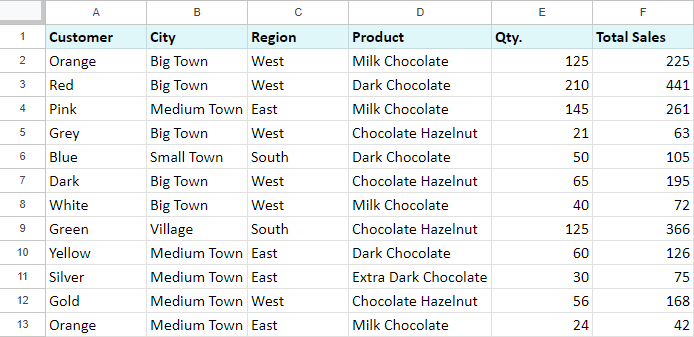Let's begin with the basics.

We need to count the number of "Milk Chocolate" sold. Place the cursor in the cell where you want to get the result and enter the equality sign (=). Google Sheets immediately understands that we are going to enter a formula. As soon as you type the letter "C", it will prompt you to choose a function that begins with this letter. Select "COUNTIF".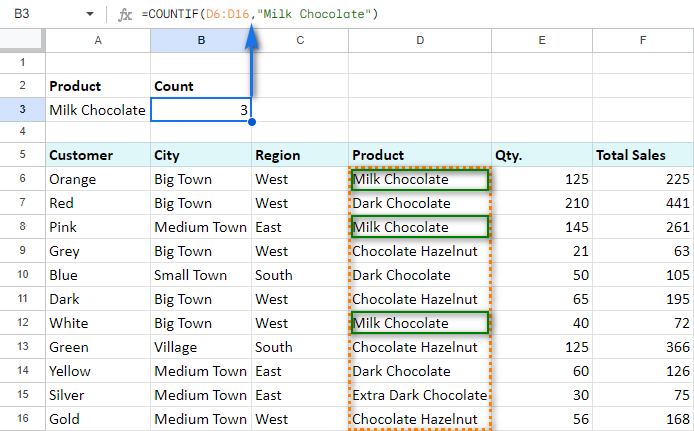The first argument of COUNTIF is represented by the following range: D6:D16. By the way, you don't have to enter the range manually - mouse selection is enough. Then enter a comma (,) and specify the second argument - searching criteria.

The second argument is a value that we're going to look for across the selected range. In our case it's going to be the text - "Milk Chocolate". Remember to finish the function with a closing bracket ")" and press "Enter".

Also, don't forget to enter double quotes ("") when using text values.

Our final formula looks as follows:

`=COUNTIF(D6:D16,"Milk Chocolate")`

As a result, we get three sales of this type of chocolate.

Note. COUNTIF function works with a single cell or neighboring columns. In other words, you can't indicate a few separate cells or columns and rows. Please see the examples below.

Incorrect formulas:

`=COUNTIF(C6:C16, D6:D16,"Milk Chocolate")`

`=COUNTIF(D6, D8, D10, D12, D14,"Milk Chocolate")`

Correct usage:

`=COUNTIF(C6:D16,"Milk Chocolate")`

`=COUNTIF(D6,"Milk Chocolate") + COUNTIF(D8,"Milk Chocolate") + COUNTIF(D10,"Milk Chocolate") + COUNTIF(D12,"Milk Chocolate") + COUNTIF(D14,"Milk Chocolate")`

You may have noticed that it's not really convenient to set the searching criteria in the formula - you have to edit it every time. The better decision would be to write the criteria down other Google Sheets cell and reference that cell in the formula.

Let's count the number of occurred sales in the "West" region using the cell reference in COUNTIF. We'll get the following formula:

`=COUNTIF(C6:C16,A3)`

The function uses the content of A3 (the text value "West") in its calculations. As you can see, it's a lot easier now to edit the formula and its searching criteria.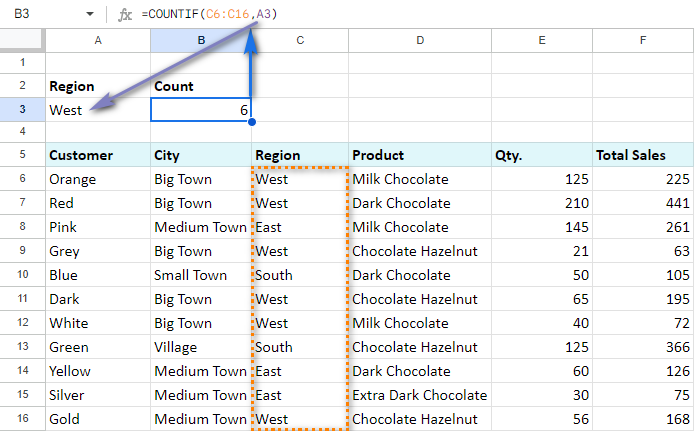Of course, we can do the same thing with numerical values. We can count the number of occurrences of the number "125" by indicating the number itself as a second argument:

`=COUNTIF(E7:E17,125)`

or by replacing it with a cell reference:

`=COUNTIF(E7:E17,A3)`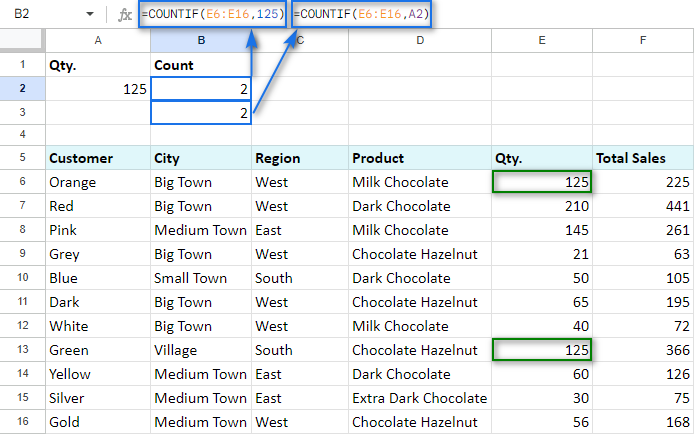### Google Spreadsheet COUNTIF function and wildcard characters (partial match)

What is great about COUNTIF is that it can count whole cells as well as parts of the cell's contents. For that purpose, we use wildcard characters: "?", "*".

For instance, to count the sales in some particular region we can use only the part of its name: enter "?est" into B3. A question mark (?) replaces one character. We are going to look for the 4-letter words ending with "est", including spaces.

Use the following COUNTIF formula in B3:

`=COUNTIF(C7:C17,A3)`

As you already know, the formula can easily take the next form:

`=COUNTIF(C7:C17, "?est")`

And we can see 5 sales in the "West" region.

Now let us employ the B4 cell for another formula:

`=COUNTIF(C7:C17,A4)`

What is more, we'll change the criteria to "??st" in A4. It means that now we are going to look for 4-letter words ending with "st". Since in this case two regions ("West" and "East") satisfy our criteria, we will see nine sales: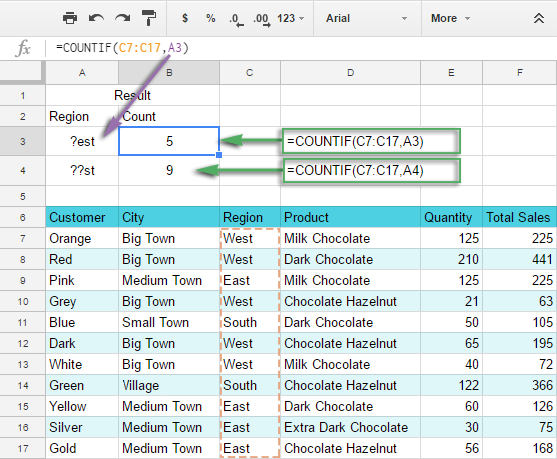Similarly, we can count the number of sales of the goods using an asterisk (*). This symbol replaces not just one, but any number of characters: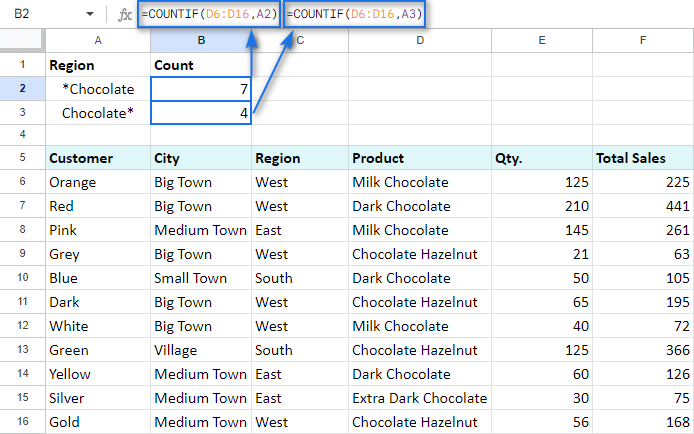"*Chocolate" criteria counts all the products ending with "Chocolate".

"Chocolate*" criteria counts all the products starting with "Chocolate".

And, as you may guess, if we enter "*Chocolate*", we're going to look for all the products that contain the word "Chocolate".

Note. If you need to count the number of words that contain an asterisk (*) and a question mark (?), then use tilde sign (~) before those characters. In this case, COUNTIF will treat them as simple signs rather than searching characters. For example, if we want to look for the values that contain "?", the formula will be:

`=COUNTIF(D7:D15,"*~?*")`

### COUNTIF Google Sheets for less than, greater than or equal to

The COUNTIF function is able to count not only how many times some number appears, but also how many of the numbers are greater than/less than/equal to/not equal to another specified number.

For that purpose, we use corresponding mathematical operators: "=", ">", "<", ">=", "<=", "<>".

Check out the table below to see how it works:

 Criteria Formula example Description The number is greater than `=COUNTIF(F9:F19,">100")` Count cells where values are greater than 100. The number is less than `=COUNTIF(F9:F19,"<100")` Count cells where values are less than 100. The number equals to `=COUNTIF(F9:F19,"=100")` Count cells where values equal to 100. The number is not equal to `=COUNTIF(F9:F19,"<>100")` Count cells where values are not equal to 100. The number is greater than or equal to `=COUNTIF(F9:F19,">=100")` Count cells where values are greater than or equal to 100. The number is less than or equal to `=COUNTIF(F9:F19,"<=100")` Count cells where values are less than or equal to 100.

Note. It's very important to enclose the mathematical operator along with a number in the double quotes.

If you want to change the criteria without altering the formula, you can reference the cells as well.

Let us reference A3 and put the formula in B3, just as we did before:

`=COUNTIF(F9:F19,A3)`

To create more sophisticated criteria, use an ampersand (&).

For example, B4 contains a formula which counts the number of values greater than or equal to 100 in the E9:E19 range:

`=COUNTIF(E9:E19,">="&A4)`

B5 has the very same criteria, but we reference not only the number in that cell but also a mathematical operator. This makes it even easier to adapt COUNTIF formula if necessary:

`=COUNTIF(E9:E19,A6&A5)`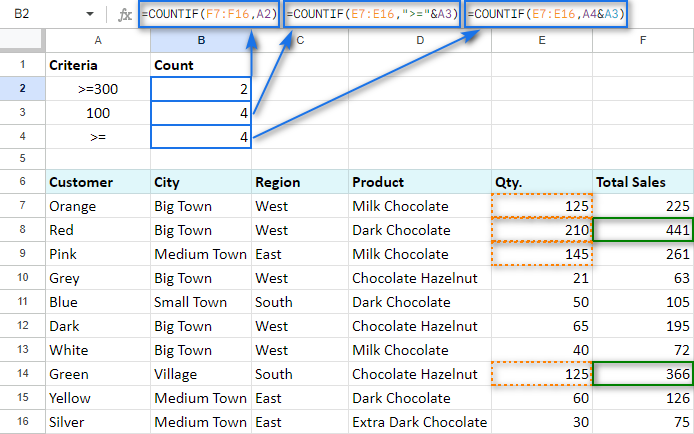Tip. We've been asked a lot about counting those cells that are greater than or less than values in another column. If that's what you're looking for, you will need another function for the job — SUMPRODUCT.

For example, let's count all rows where sales in column F are bigger than in the same row of column G:

`=SUMPRODUCT(--(F6:F16>G6:G16))`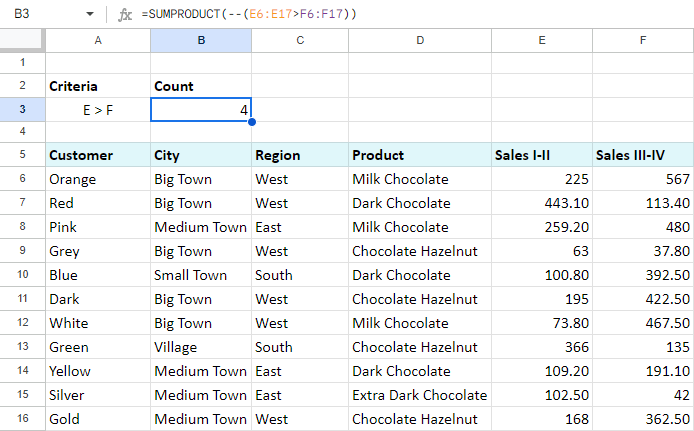• The part at the core of the formula — F6:F16>G6:G16 — compares values in columns F and G. When the number in column F is greater, the formula takes it as TRUE, otherwise — FALSE.

You'll see that if you enter the same into the ArrayFormula:

`=ArrayFormula(F6:F16>G6:G16)`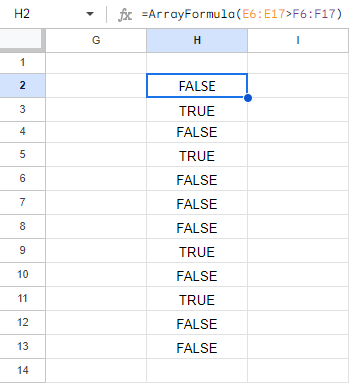• Then the formula takes this TRUE/FALSE result and turns it into 1/0 numbers with the help of the double unary operator (--).
• This lets SUM do the rest — total the number of when F is greater than G.

## Google Spreadsheet COUNTIF with multiple criteria

Sometimes it's necessary to count the number of values that answer at least one of the mentioned conditions (OR logic) or multiple criteria at once (AND logic). Based on that, you can use either a few COUNTIF functions in a single cell at a time or the alternate COUNTIFS function.

### Count in Google Sheets with multiple criteria — AND logic

The only way I’d advise you to use here is with a special function that is designed to count by multiple criteria — COUNTIFS:

=COUNTIFS(criteria_range1, criterion1, [criteria_range2, criterion2, ...])

It is normally used when there are values in two ranges that should meet some criteria or whenever you need to get the number falling between a specific range of numbers.

Let’s try and count the number of total sales between 200 and 400:

`=COUNTIFS(F8:F18,">=200",F8:F18,"<=400")`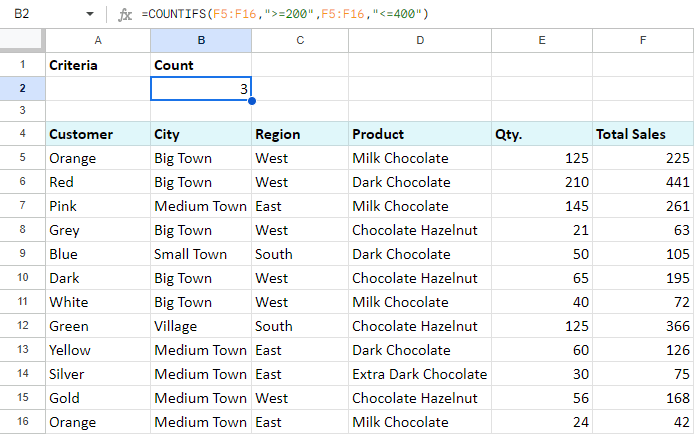### Count uniques in Google Sheets with multiple criteria

You can go further and count the number of unique products between 200 and 400.

Nope, it's not the same as above! :) The above COUNTIFS counts each occurrence of sales between 200 and 400. What I suggest is to also look at the product. If its name occurs more than once, it won't be included in the result.

There's a special function for that — COUNTUNIQUEIFS:

COUNTUNIQUEIFS(count_unique_range, criteria_range1, criterion1, [criteria_range2, criterion2, ...])

Compared to COUNTIFS, it's the first argument that makes the difference. Count_unique_range is that range where the function will count unique records.

Here's how the formula and its result will look:

`=COUNTUNIQUEIFS(D6:D16,F6:F16,">=200",F6:F16,"<=400")`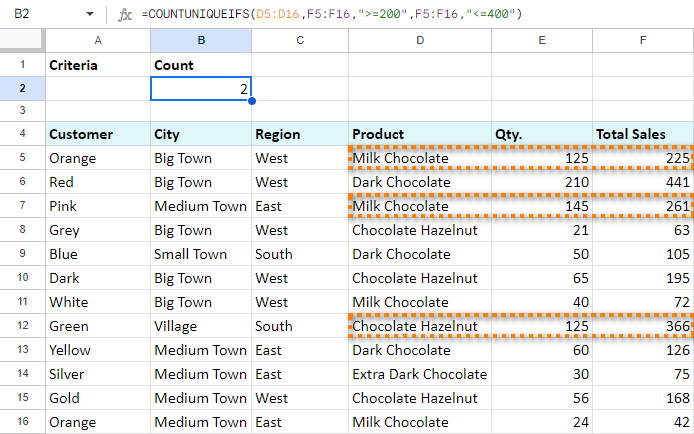Look, there are 3 rows that meet my criteria: the sales are 200 and greater and at the same time are 400 or less.

However, 2 of them belong to the same product — Milk Chocolate. COUNTUNIQUEIFS counts the first mention of the product only.

Thus, I know that there are only 2 products that meet my criteria.

### Count in Google Sheets with multiple criteria — OR logic

When only one of all criteria is enough, you’d better use several COUNTIF functions.

#### Example 1. COUNTIF + COUNTIF

Let's count the number of sales of black and white chocolate. To do that, enter the following formula in B4:

`=COUNTIF(D7:D17,"*Milk*") + COUNTIF(D7:D17,"*Dark*")`

Tip. I use asterisk (*) to ensure that the words "dark" and "milk" will be counted no matter where they are in the cell — at the beginning, in the middle, or at the end.

Tip. You can always introduce cell references to your formulas. See how it looks on the screenshot below in B3, the result remains the same: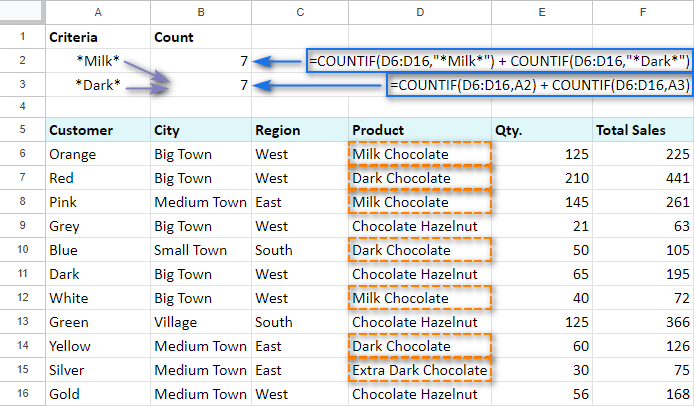#### Example 2. COUNTIF — COUNTIF

Now, I am going to count the number of total sales between 200 and 400: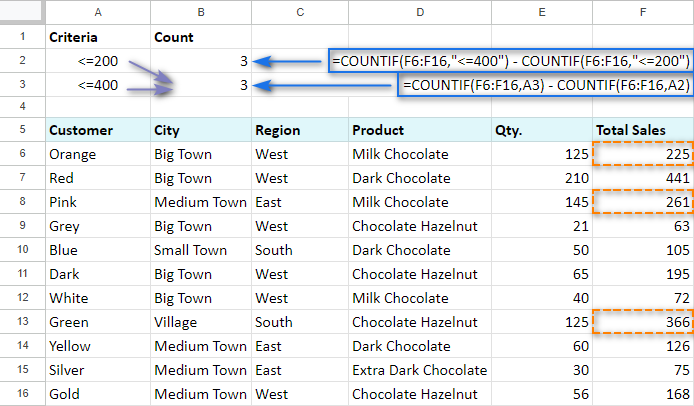I take the number of totals under 400 and subtract the number of total sales under 200 using the next formula:

`=C0UNTIF(F7:F17,"<=400") - COUNTIF(F7:F17,"<=200")`

The formula returns the number of sales more than 200 but less than 400.

If you decide to reference A3 and A4 that contain the criteria, the formula will be a bit simpler:

`=COUNTIF(F7:F17, A4) - COUNTIF(F7:F17, A3)`

A3 cell will have "<=200" criteria, while A4 - "<=400". Put both formulas into B3 and B4 and make sure that the result doesn't change — 3 sales over the needed range.

## COUNTIF Google Sheets for blank and non-blank cells

With the help of COUNTIF, we can also count the number of blank or non-blank cells within some range.

Let's suppose that we successfully sold the product and marked it as "Paid". If the customer declined the goods, we write zero (0) in the cell. If the deal wasn't closed, the cell remains empty.

To count non-blank cells with any value, use the following:

`=COUNTIF(F7:F15,"<>")`

or

`=COUNTIF(F7:F15,A3)`

To count the number of empty cells, make sure to put the COUNTIF formula in the following way:

`=COUNTIF(F7:F15,"")`

or

`=COUNTIF(F7:F15,A4)`

The number of cells with a textual value is counted like this:

`=COUNTIF(F7:F15,"*")`

or

`=COUNTIF(F7:F15,A5)`

Screenshot below shows that A3, A4, and A5 cells include our criteria: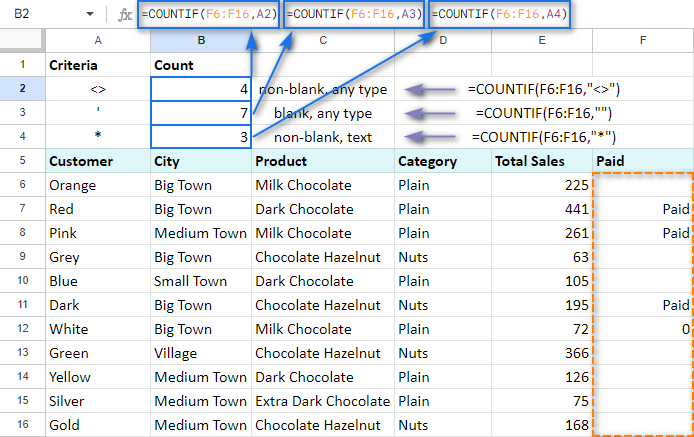Thus, we can see 4 closed deals, 3 of which were paid for and 5 of which have no markings yet and, consequently, are not closed.

## COUNTIF and conditional formatting

There is one interesting opportunity that Google Sheets offer - to change the cell's format (like its color) depending on some criteria. For example, we can highlight the values that appear more often in green.

COUNTIF function can play a small part here as well.

Select the range of the cells that you want to format in some special way. Click Format -> Conditional formatting...

In the Format cells if... drop-down list choose the last option Custom formula is, and enter the following formula into the appeared field:

`=COUNTIF(\$B\$10:\$B\$39,B10)/COUNTIF(\$B\$10:\$B\$39,"*")>0.4`

It means that the condition will be answered if the value from B10 appears within B10:B39 in more than 40% of cases: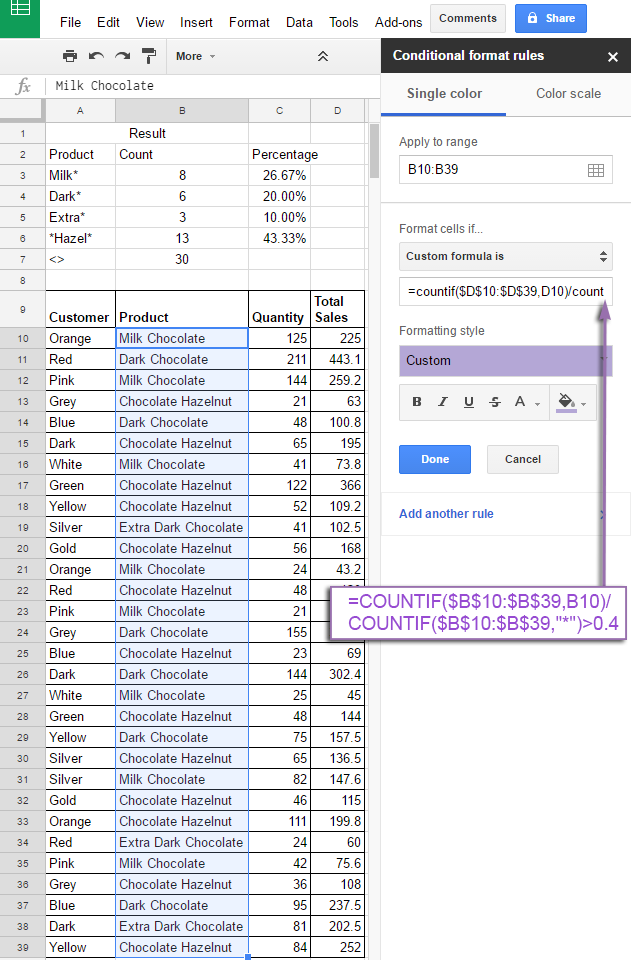In a similar way, we add two more formatting rule criteria - if the cell value appears more often than in 25% of cases and more often than in 15%:

`=COUNTIF(\$B\$10:\$B\$39,B10)/COUNTIF(\$B\$10:\$B\$39,"*")>0.25`

`=COUNTIF(\$B\$10:\$B\$39,B10)/COUNTIF(\$B\$10:\$B\$39,"*")>0.15`

Keep in mind that the first criterion will be checked beforehand, and if it's met, the rest won't apply. That is why you'd better start with the most unique values moving to the most common ones. If the cell value doesn't meet any criteria, its format will remain intact.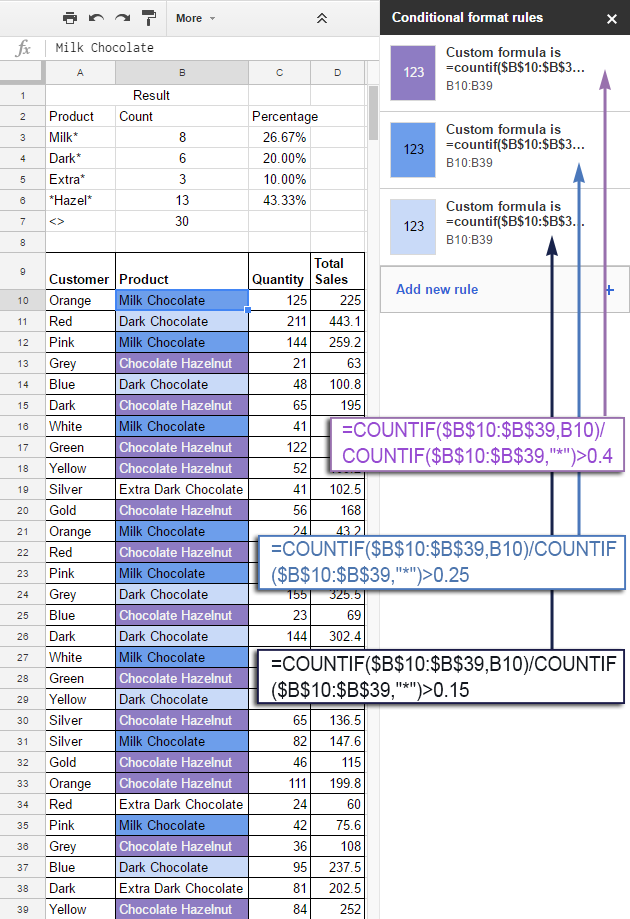You can see that the colour of the cells has changed according to our criteria.

To make sure, we also counted the frequency of some values in C3:C6 using COUNTIF function. The results confirm that COUNTIF in formatting rule was applied correctly.

All these function examples give us a clear understanding of how Google Spreadsheet COUNTIF offers multiple opportunities to work with the data in a most efficient way.